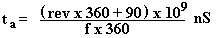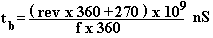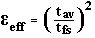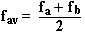# Characteristic Impedance Calculator

Calculates Delay, Velocity Factor, Effective Dielectric Constant and Characteristic Impedance of a PCB track. This calculator can also be used for measuring coaxial cable and will then give Relative Dielectric Constant rather than Effective Dielectric Constant. The operation of this calculator can be better understood by first reading Measuring Characteristic Impedance and Effective Dielectric Constant of PCB tracks using a Vector Network Analyser

 INPUT DATA Frequency at + 900 (fa) : MHz SHORT R: Ω SHORT J: Ω OPEN R: Ω OPEN J: Ω Frequency at - 900 (fb) : MHz SHORT R: Ω SHORT J: Ω OPEN R: Ω OPEN J: Ω Complete Phase Revolutions: Mechanical Length: mm
 RESULTS Delay( tav): ns Velocity Factor: Effective Dielectric Constant (εeff): Impedance Real (R): Ω Imaginary (j): Ω Characteristic Impedance (Zo): Ω Averaged Frequency: MHz

This calculator uses JavaScript and will function in most modern browsers. For more information see About our calculators

The time delay (ta) at a + 900 point is found by dividing the total phase shift at the measured + 900 point by the phase shift per second due to frequencyFormula 1.

Where f is the frequency in Hz at + 900

rev is the number of completed revolutions of phase before the + 900 point is reached

Similarly the time delay (tb) at a - 900 point is found by dividing the total phase shift at the measured - 900 point by the phase shift per second due to frequencyFormula 2.

The average time delay (tav) for + 900  and - 900 is thenFormula 3.

Finding the Velocity Factor

Equivalent delay in free space for a similar distanceFormula 4.

Where l is the length of the track in mm

c is the speed of light in free spaceFormula 5.Formula 6.

An average of the measurement frequencies is foundFormula 7.

The principal of deriving the Characteristic Impedance from an OPEN and SHORT measurement is based on Transmission Lines and Networks by Walter C. Johnson, McGraw-Hill 1963 p155 Para 4.7 Measurement of the Characteristics of lines.Formula 8

This calculation is combined for two adjacent measurements and so ripple (measurement error) caused by the mismatch of the VNA to the transmission line is also removed.Formula 9.

W J Highton Updated 4/4/2008

This calculator is provided free by Chemandy Electronics in order to promote the FLEXI-BOX

Return to Calculator Index